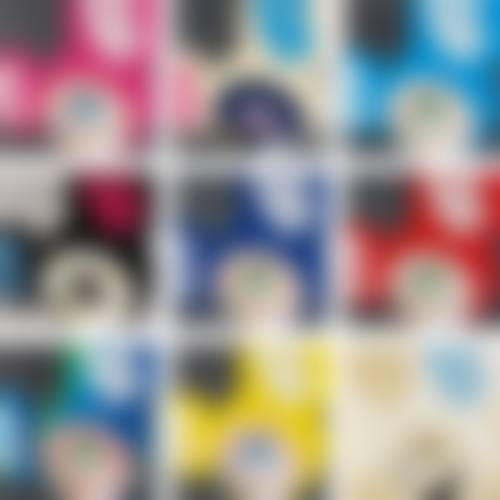##### And Then And Then And Then And Then And Then (Pink); And Then Platinum; And Then And Then And Then And Then And Then (Aqua Blue); And Then Black; And Then And Then And Then And Then And Then (Blue); And Then And Then And Then And Then And Then (Red); Melting Dob A; And Then And Then And Then And Then And Then (Yellow); And And Then Ichimatsu PatternSell Through Rate: 100%

Artwork: And Then And Then And Then And Then And Then (Pink); And Then Platinum; And Then And Then And Then And Then And Then (Aqua Blue); And Then Black; And Then And Then And Then And Then And Then (Blue); And Then And Then And Then And Then And Then (Red); Melting Dob A; And Then And Then And Then And Then And Then (Yellow); And And Then Ichimatsu Pattern
Size: all sheet: 68 x 68 cm
Edition Size: 300
Medium: Offset lithographs in colours on smooth wove paper the full sheets
Creation year: 2006
Category: Prints
Artwork details : Set of 9, published by Kaikai Kiki Co., Ltd., Tokyo, created 1999-2006

### Phillips London

Date: 2016-06-09
Lot Number: 237
Notes: All signed and numbered variously in black or silver ink, all framed